T-SQL

# Updating LOBs using the WRITE() column method

The WRITE (expression,@Offset,@Length) extension of the UPDATE statement can be used to perform updates that insert or append new data in minimally logged mode if the database recovery model is set to bulk-logged or simple. Minimal logging is not used when existing values are updated. This all is valid for large data types varchar(max), nvarchar(max), and varbinary(max) . Let me in short demonstrate how it works and the most interesting part of this post is an evaluation of the real performance gain we can get when using the WRITE() instead of the traditional approach like concatenation or REPLACE().

We will review the basic usage of the WRITE() extension first. Let’s create a sample table and insert the ‘INITIAL_VALUE’ test string:

```DROP TABLE IF EXISTS dbo.SampleTable

CREATE TABLE dbo.SampleTable (Val NVARCHAR(MAX) NOT NULL)

INSERT INTO [dbo].[SampleTable] ( Val ) VALUES ( N'INITIAL_VALUE')

SELECT * FROM [dbo].[SampleTable]
GO```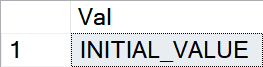Contents

##### Append

The easiest operation we can do with the WRITE() is appending a new string to the end of the existing one:

`UPDATE dbo.[SampleTable] SET [Val].WRITE('AAAA_BBBB_CCCC_DDDD', NULL, NULL)`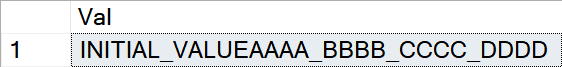##### Replace

When we will set the @Offset value to 0 and @Length will be NULL the WRITE() will perform a full string replacement operation:

`UPDATE dbo.[SampleTable] SET [Val].WRITE('AAAA_BBBB_CCCC_DDDD', 0, NULL)`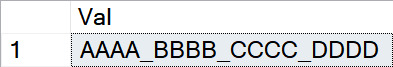##### Inject

When the @Offset is used and @Length is set to 0 then the expression is inserted at the @Offset position inside an existing value:

`UPDATE dbo.[SampleTable] SET [Val].WRITE('AAAA_BBBB_CCCC_DDDD', 8, 0)`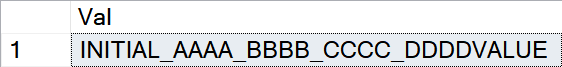##### Cut

If we would like to cut an exact part (@Length) of the value at a certain position (@Offest):

```UPDATE dbo.[SampleTable] SET [Val] = 'AAAA_BBBB_CCCC_DDDD'
UPDATE dbo.[SampleTable] SET [Val].WRITE('', 5, 5)```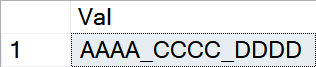##### Erase

When we need to make the LOB column value to be empty (remember, it can’t be NULL when WRITE() is used) below-listed combination of parameters can be used:

```UPDATE dbo.[SampleTable] SET [Val].WRITE(NULL, 0, 0)
```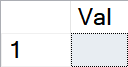### Performance check

The following script will perform two + two updates of the [Val] column. The first pair will use the traditional approach (value assignment and REPLACE()) and the second one is doing exactly the same using the WRITE() method. Updates are executed in a total of 100k cycles because for a single update the performance difference is hard to be measured. You can change the number of batches as needed based on your local system performance.

```SET NOCOUNT ON

DECLARE @a NVARCHAR(MAX)
DECLARE @b NVARCHAR(MAX)
DECLARE @c NVARCHAR(MAX)
DECLARE @x NVARCHAR(MAX)

SET @a = REPLICATE(CAST('a' AS NVARCHAR(MAX)), 500)
SET @b = REPLICATE(CAST('b' AS NVARCHAR(MAX)), 1000)
SET @c = REPLICATE(CAST('c' AS NVARCHAR(MAX)), 500)
SET @x = REPLICATE(CAST('x' AS NVARCHAR(MAX)), 1000)

DECLARE @i INT = 100000

DECLARE @ST DATETIME2

SET @ST = SYSDATETIME()

WHILE @i > 0
BEGIN

UPDATE [dbo].[SampleTable] SET [Val] = @a + @b + @c
UPDATE [dbo].[SampleTable] SET [Val] = REPLACE([Val], @b, @x)

SET @i = @i -1

END

SELECT DATEDIFF(ms, @ST, SYSDATETIME()) AS [Standard]

SET @ST = SYSDATETIME()

SET @i = 100000

WHILE @i > 0
BEGIN

UPDATE [dbo].[SampleTable] SET [Val].WRITE(@a + @b + @c, 0, NULL)
UPDATE [dbo].[SampleTable] SET [Val].WRITE(@x, 500, 1000)

SET @i = @i -1

END

SELECT DATEDIFF(ms, @ST, SYSDATETIME()) AS [WRITE]
GO```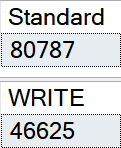There is a nearly 50% performance gain when the WRITE() method is used to update LOB column instead of the traditional approach.

It’s worth mentioning that the performance gain heavily depends on the size of the strings used for testing. The larger the strings are, the bigger is the performance gain. When we will divide all string lengths from our test case by 10, the performance gain will be only about 17% (47 vs 40 seconds). If we will multiply them by 4 then the gain will grow to about 330%! (177 vs 53 seconds). This is an amazing improvement.

### Overriding the REPLACE() limitation

There is a known limitation for the REPLACE ( string_expression , string_pattern , string_replacement ) function: The string_pattern cannot exceed the maximum number of bytes that fits on a page. You can simply test it. Remember that the 4001 value is there because the Unicode NVARCHAR() datatype is used. Otherwise, 8000 will be the maximum allowed length.

```DECLARE @a NVARCHAR(MAX);
DECLARE @b NVARCHAR(MAX);
DECLARE @c NVARCHAR(MAX);
SET @a = REPLICATE(CAST('a' AS NVARCHAR(MAX)), 10000);
SET @b = REPLICATE(CAST('b' AS NVARCHAR(MAX)), 4001);
SET @c = REPLICATE(CAST('c' AS NVARCHAR(MAX)), 10000);

SELECT REPLACE(@a + @b, @b, @c);
GO```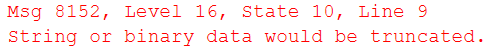This limitation makes it obvious that the REPLACE() function can’t be used when we need to adjust really large strings. There is no such limitation for the WRITE() method. That all should point you to remember that the WRITE() can be your good friend from time to time.